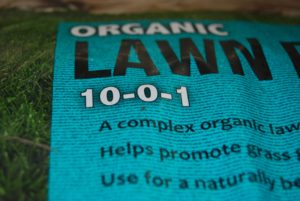# Fertilizer Calculator

How much fertilizer should you apply based on the square footage of your lawn and your soil test results? Use the Lawn to Lake Fertilizer Calculator below to find out.

Calculator

### Want to know where these numbers came from?The N-P-K ratio on a fertilizer bag looks like this. | Photo Credit: Janice Milanovich, Illinois-Indiana Sea Grant

If you’d like to calculate the total pounds of fertilizer needed yourself, you can. For example, let’s figure out how much fertilizer you need to apply a fertilizer with a N-P-K ratio of 22-0-15 at 1 pound (lb) of Nitrogen (N) per 1,000 square foot (ft2) to a 5,000 ft 2 lawn.

• First, to calculate the total fertilizer need (in lbs. per 1, 000 ft2), divide the desired rate (lbs. N per 1, 000 ft2) by the % nutrient.
• 1 /1,000 ft2 divided by .22 = 4.545, round to 4.5 lbs./1,000 ft2
• Next, using that information, you can calculate the pounds of fertilizer needed for your sized lawn. Multiply the total fertilizer need from the first calculation (lbs./1, 000 ft2) by the size of the area to be treated (ft2).
• 4.5 lbs./1,000 ft2 multiplied by 5,000 ft2 = 22.5 lbs
• Therefore, 22.5 lbs. of fertilizer are needed for a 5,000 ft2